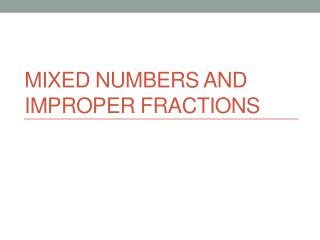# Mixed Numbers and Improper Fractions - PowerPoint PPT PresentationDownload PresentationMixed Numbers and Improper Fractions

Mixed Numbers and Improper Fractions
Download Presentation## Mixed Numbers and Improper Fractions

- - - - - - - - - - - - - - - - - - - - - - - - - - - E N D - - - - - - - - - - - - - - - - - - - - - - - - - - -
##### Presentation Transcript

1. Mixed Numbers and Improper Fractions

2. NS 2.1 Solve problems involving addition, subtraction, multiplication, and division of positive fractions and explain why a particular operation was used for a given situation. • Today’s objective: learn how to convert between mixed numbers and improper fractions • Learning target: Convert at least 3 of the 4 mixed numbers and improper fractions correctly on the exit ticket.

3. A number with an integer and a proper fraction. • Examples: • Non-examples: • What is a mixed number?

4. A fraction whose numerator is greater than the denominator. • Examples: • Non-examples: • What is an improper fraction?

5. When you need to see how many whole parts there are. • When we are multiplying or dividing two amounts. • When is it useful to use mixed numbers? • When is it useful to use improper fractions?

6. How do we convert mixed numbers into improper fractions?

7. Let’s slice up all 4 wholes into 8 pieces each to match the fraction at the end. How many pieces do we have now? The 4 wholes each became 8 pieces, so there are 4×8 = 32 pieces from those. Then add the 7 pieces we started with: 32 + 7 = 39 pieces. It still takes 8 pieces to form a whole circle, so the denominator remains 8.

8. Multiply the denominator by the whole number and add the numerator to get the new numerator. The denominator remains the same. • How do we convert mixed numbers into improper fractions?

9. Convert into improper fractions:

10. How do we convert improper fractions into mixed numbers?

11. How many wholes can we make out of these pieces? Since the denominator is 4, we need 4 pieces to form one whole. To find how many groups of 4 there are in 13, do the long division: We can form 3 whole circles and will have 1 out of the 4 pieces required to make another circle.

12. Divide the numerator by the denominator. The quotient is the whole number, the remainder is the new numerator, and the denominator remains the same. • How do we convert improper fractions into mixed numbers?

13. Convert into mixed numbers:

14. Direct Station • We will do word problems that involve real-life situations that require converting between mixed numbers and improper fractions.

15. Collaborative Station • We will play the card game “War” in which each player flips over a card and whoever’s number is greater adds both cards to the bottom of their deck. • Write down the work showing how you figured out whose number was greater. • The goal is to get all of the cards in your deck.

16. Collaborative Station Example • The cards are evenly divided between Partners A and B. They each hold their decks face down. • Both partners flip over the top card of their deck. Partner A’s card is 7/2 and Partner B’s card is 4 1/2. • The partners calculate that 7/2 = 3 ½ OR The partners calculate that 4 ½ = 9/2. • Partner B’s card is worth more so he puts both cards on the bottom of the deck. • If for one round you compared by turning the mixed number into an improper fraction, for the next round turn the improper fraction into a mixed number instead.

17. Independent Station • We are continuing ST Math’s unit on fraction addition and subtraction. • Make sure you are writing down your calculations.